## 微积分的历史（四），发展之伯努利兄弟1 链式法则

$ln(sin(x))=ln'(sin(x))sin'(x)=\frac{1}{sin(x)}cos(x)$

《高等数学》同济版

1.1 通过物理模型来理解链式法则

Created with GeoGebra

1.2 理解误区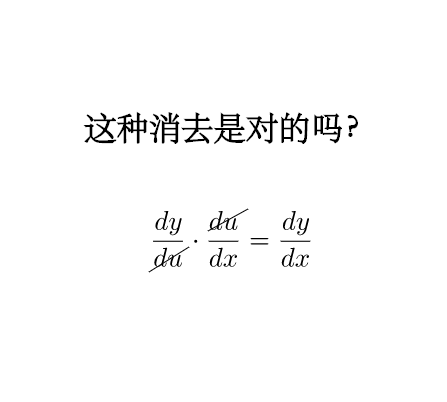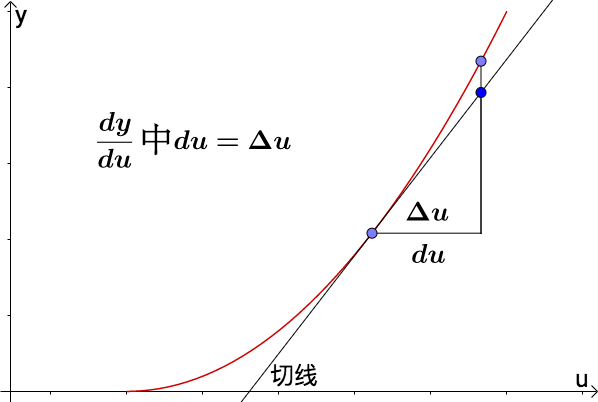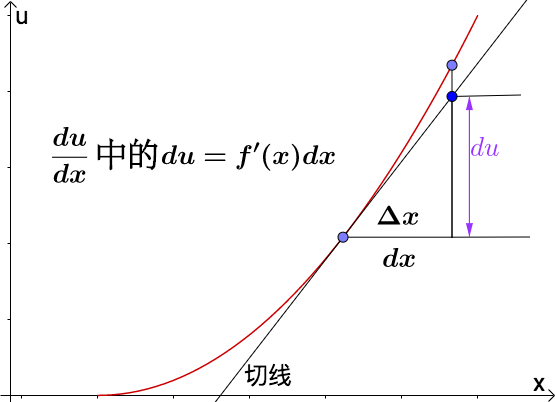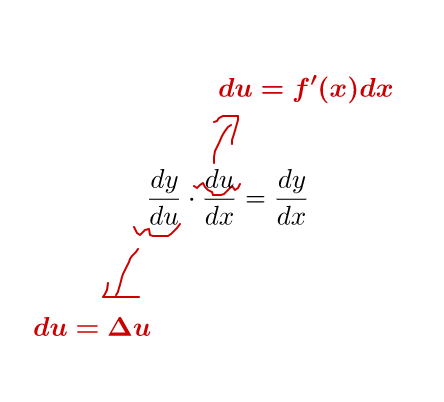1.2.1 一阶微分不变性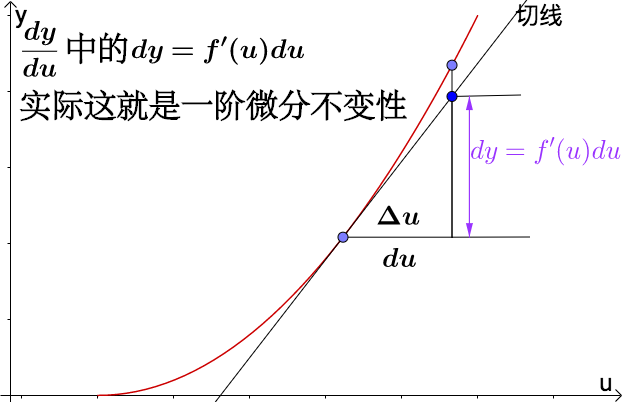1.3 小结

2 洛必达法则

2.1 定义

2.2 切线方程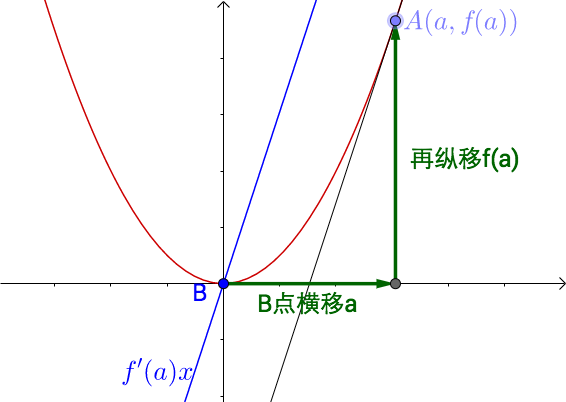2.3 洛必达法则与线性逼近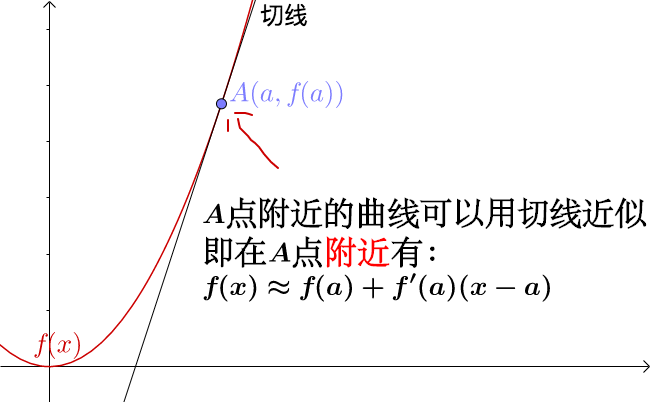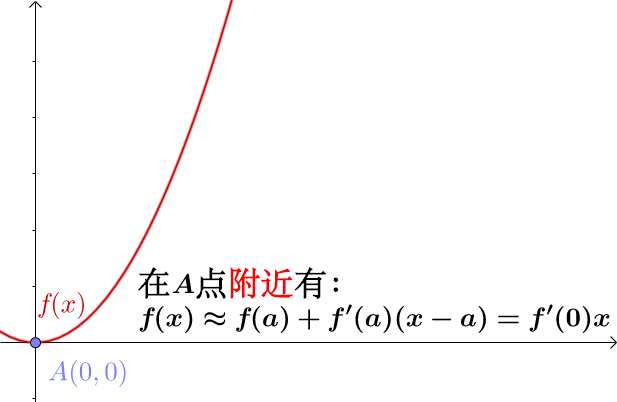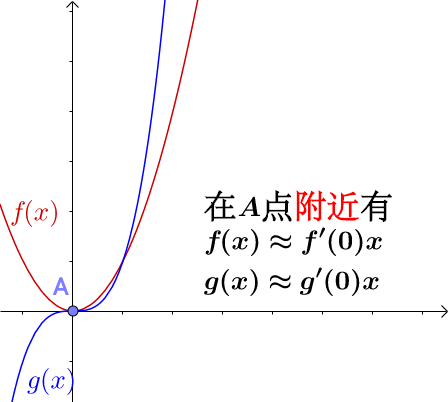$$\frac{f(x)}{g(x)}\approx \frac{f'(0)x}{g'(0)x}=\frac{f'(0)}{g'(0)}\\ \implies \frac{f(x)}{g(x)}\approx \frac{f'(0)}{g'(0)}$$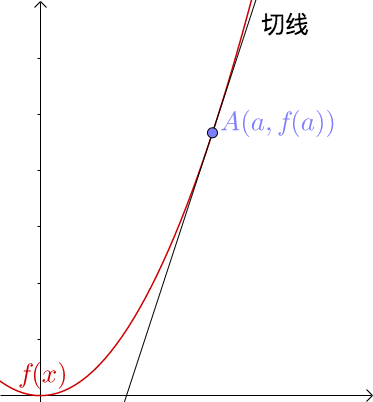$$\displaystyle \lim _{x\to 0}\frac{f(x)}{g(x)}=\frac{f'(0)}{g'(0)}$$

$$\displaystyle \lim _{x\to 0}\frac{f(x)}{g(x)}=\lim _{x\to 0}\frac{f'(x)}{g'(x)}$$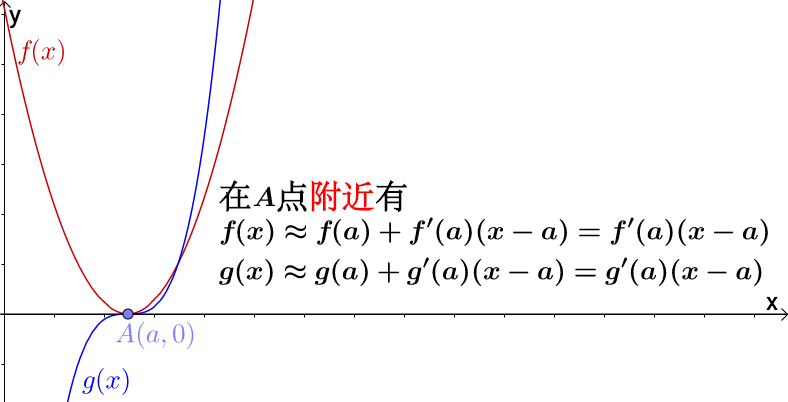$$\frac{f(x)}{g(x)}\approx \frac{f'(a)}{g'(a)}$$

$\frac{f(x)}{g(x)}\implies \frac{\frac{1}{g(x)}}{\frac{1}{f(x)}}$，$\frac{1}{f(x)}$和$\frac{1}{g(x)}$又是$0/0$型了。

2.4 小结

3 无穷级数

3.1 数学的基本元素

$\displaystyle \int {x^ xdx} = \int {e^{\ln x^ x}dx} = \int {\sum _{k=1}^{\infty }\frac{x^ k\ln ^ k x}{k!}}dx$

3.2 积分的基础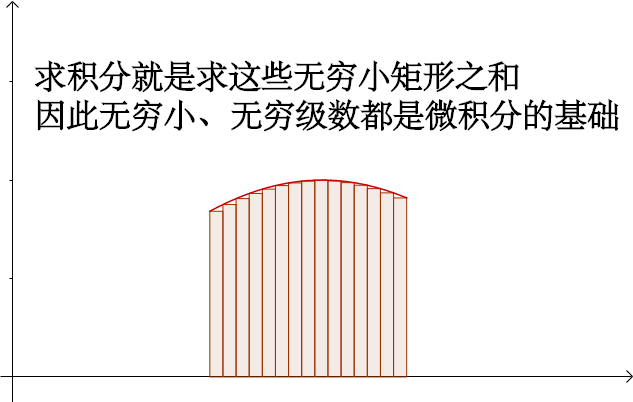3.3 泰勒级数